Square
 To the Main Page  "Mathematische Basteleien"

What is a Square?
 ...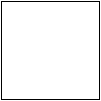. A square is a four-sided figure with four right angles and four equal sides.

 ....... The same figure stands on one corner.  It is called a diamond to express the new position of the square.

Variables of the Square    top
The square has four equal sides.
Two sides meet in one corner and are perpendicular to each other.
 ...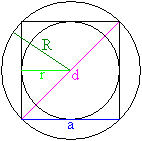... The length of a side is a.  The square has two diagonals of the length d=a*sqr(2).  The area is A=a², the perimeter is U=4a. The circumscribed circle has the radius R=sqrt(2)/2*a, the inscribed circle the radius  r=a/2.

Only one Formula top
 ...It is possible to describe a square by only one formula in a coordinate system.    |x|+|y|=2 or abs(x)+abs(y)=2

Square Numbers   top
You get square numbers if you multiply natural numbers by themselves.
 ...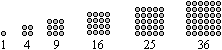... The square numbers can be illustrated by squares.

 ...Each square number is the sum of two triangular numbers. Formula: n²=[1+2+3+...+n] + [1+2+3+...+(n-1)]

 ...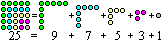Each square number n² is the sum of the n first odd numbers. Formula: n²=1+3+5+...+(2*n-1)

 ...You can divide a square into two smaller squares and two equal rectangles.  There is the first binomial theorem (a+b)²=a²+2*a*b+b².

 ...... You can divide a square into strips in a diagonal direction.  There is 1+2+3+...+n+...3+2+1=n².Divide a Square in Half top
There are many possibilities to halve the area of a square. Here are five.To 1 and 2: The common way is dividing the square by a diagonal or a median. So you can fold a square sheet of paper.
To 3: Each straight line going through the centre of a square cuts it in two congruent trapeziums.
To 4: You can also use other lines like half circles instead of straight lines.
To 5: The figure in the middle consists of four congruent triangles, the whole figure of  8 ones. So the halving is clear. If the start square has the area 2, green square has the area 1 and the length sqrt(2). The importance of this figure is the fact that the outer square has a rational, the inner one an irrational length.

Square inside a Square top
 ....... If you measure a line (red) starting in a corner and going in one direction you get four points. If you connect them you get a square.  Proof: The four triangles are congruent because of the theorem side/angle/side. The angle is the right angle in the middle. Thus the hypotenuses are equal and they are the sides of the figure. Its angles are 90°.

Square and Circle  top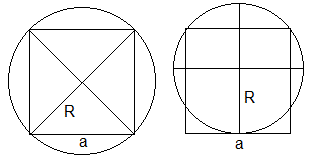In the drawing on the right, a circle goes through all four corners of a square.  This is the circumcircle with R=(1/2)sqrt(2)a or approximately 0.7a. In the left drawing a circle goes through two corners and touches one side. According to the geometric mean theorem, the radius is R=(5/8)a or about 0.6a.

Sequence of Squares top
 ...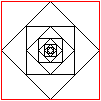... The red square has the side length a and thus the area a².  One the other side there is the sum of all inner squares:  A= a²/2 + a²/4+a²/8+a²/16+... = a²(1/2+1/4+1/8+1/16+...).  The series in brackets is geometric. It has the limit 1.
Result: The sum of the areas of the inner squares approaches the area of the whole square.

The binomial and the Pythagorean theorems use squares.
You find more on my page Illustrations of Formulas

Tiling the Square  top
It is easy to divide a square in equal squares. It is difficult to find different squares which can do that.
 ...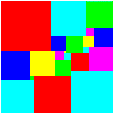... You need at least 21 different squares which form a square (Duijvestijn 1962, 1978). Solution: 112² = 50²+42²+37²+35²+33²+29²+27²+25²+24²+19²+18²+17²+16²+15²+11²+9²+8²+7²+6²+4²+2²
Many mathematicians (and their computers) worked on that problem (Book 2). You find more on Erich Friedman's web site (URL below).

An Infinite Geometric Series   top
 ...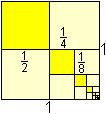The geometric progression 1/4+1/16+1/64+ ... has the limit 1/3. Proof: s=a[1/(1-r)]=(1/4)[1/(1-(1/4)]=1/3 There is a remarkable picture on the left. Found at bei http://en.wikipedia.org/wiki/The_Quadrature_of_the_Parabola

Largest and Smallest Rectangles   top
The square is a special rectangle. You can see that in the following solutions of extremum problems.
Problem 1) Which rectangle of equal perimeters has the largest area?
Problem 2) Which rectangle of equal areas has the smallest perimeter?
Problem 3) Which rectangle of an equilateral triangle has the largest area?
Problem 4) Which rectangle inside a square has the largest area?
Problem 5) Which rectangle inside a circle  has the largest area?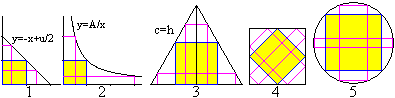In all cases a square is the searched rectangle.
You find the largest square inside a cube on my cube page.

Writing Digits  top
Matrix of squares
 ...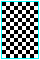.... The pixels used for writing letters of fonts form a small matrix square, which you can only see with sufficient magnification.  You use a matrix 9x14 for the picture of the digit 2 in the font "Times New Roman"of Winword. ..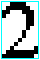....

 ...... If you double a square, you get a form, which is able to show all ten digits.  This is well known from the calculator. ......

Solomon's ring
 ...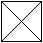.... In an old legend Lucas tells that a square with diagonals was engraved in one of Solomon's rings. The ten digits are hidden here [Book 1]. ..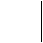....

Keyboard of a calculator
 ...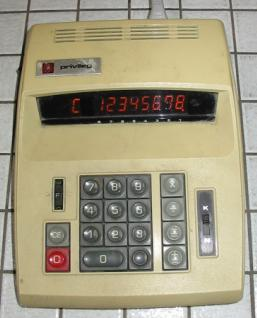... You can also take the keyboard of a calculator as an example. The numbers 1 to 9 are on square keys. Altogether they form a 3x3 square. It is strange that the numbers go from right to left and from the bottom to the top. The numbers on the keyboard of a telephone run like our writing.  I remember that people tried to find a standardized order of the keyboard for telephone and calculator. That went wrong. The first cheap calculators came from the Far East and they took their order.  Some nostalgia: I still have a calculator from 1971 (Quelle "Privileg"), which has been working until now (2004). The price was 300 DM (about 150 Euro). It used 220V for glow lamps for showing the numbers. It didn't know the rule "multiplying first, adding second" and didn't show the front zeros.
Looking back this calculator was a big help and saved much time ;-).

Optical Illusions  top
You see: The squares on the left are different in their side lengths, those on the right in their areas.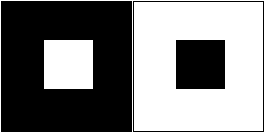You already supposed: The squares have the same measurements.

You see: Four four-sided figures lie in front of a sequence of circles, squares, or straight lines. The sides look bent.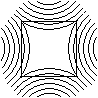You already supposed: There are congruent squares.
You see: Both grids have grey spots at the crossing points.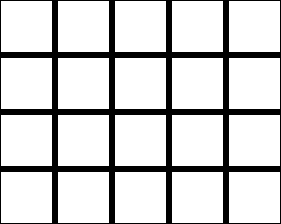You already supposed: The grey spots are imagination.

An arrangement of four 3/4 circles
 ...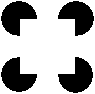There is no square drawn, in spite of that you see it.

Polyominos   top
You can arrange squares for complex figures. You demand that two squares always touch in one side.The figures are counted up to 28-ominos. Look at the last line.

This is a possible way to the Pentominos.You find more on my pages Domino Puzzle, Pentominos und Hexominos.

If you also admit 3d-figures, you get monoids.
 ...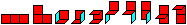... You give three squares for example. You can built eight 3d-monoids except the two triominos.

You find more on my page Rubik's Magic. There are many figures of eight squares.

There is a systematic investigation of the monoids on Jorge L. Mireles Jasso's web site (URL below).

Games and Squares top
If you look at the most common games under square views, you are successful. Nearly all games use squares in the board, the playing field, or the peg.

I only list all the games which I describe on other places of my homepage:

Squares on the Internet top

German

Wikipedia

English

Eric W. Weisstein (MathWorld)
Square, Square Packing, Perfect Square Dissection

Erich Friedman
Erich's packing center

Kevin L. Gong
counting polyominoes

Kevin L. Gong
counting polyominoes

Wikipedia
Square

References   top
(1) Walter Lietzmann: Lustiges und Merkwürdiges von Zahlen und Formen, Göttingen 1969
(2) Friedrich L. Bauer: Einladung zur Mathematik, Deutsches Museum, München 1999

Feedback: Email address on my main page